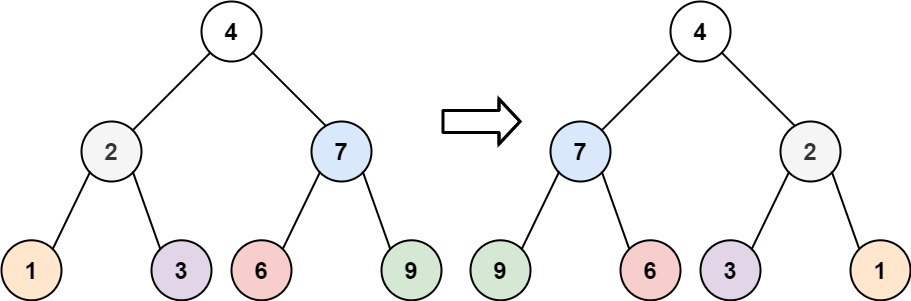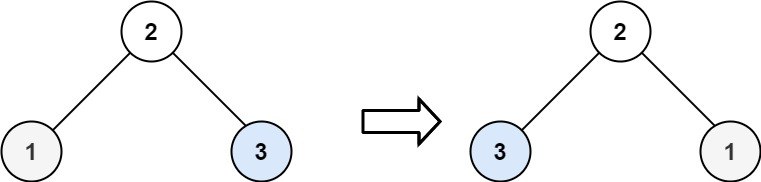# Invert Binary Tree LeetCode Solution

Difficulty Level Easy

## Problem Statement:

Invert Binary Tree LeetCode Solution : Given the `root` of a binary tree, invert the tree, and return its root.

An inverted form of a Binary Tree is another Binary Tree with left and right children of all non-leaf nodes interchanged. You may also call it the mirror of the input tree. NOTE: The tree is binary so there could be a maximum of two child nodes. To put it simply, inverting a binary tree means creating its mirror image. More formally, it means swapping the children of each of its non-leaf nodes. Therefore, the left child of a node will take the place of the right child and vice-versa.

## Examples:

Example 1:Input:

``` root = [4,2,7,1,3,6,9]
```

Output:

``` [4,7,2,9,6,3,1]
```

Example 2:Input:

``` root = [2,1,3]
```

Output:

``` [2,3,1]
```

Example 3:

Input:

``` root = []
```

Output:

` []`

## Approach:

### Idea:

We will solve the problem with the help of recursion. We only need to swap the left and right child pointers and do the same for the child pointers recursively.

One way to invert a binary tree is to use the power of recursion. Every node contains a value (in our case, an integer) and two pointers, one for each of the node’s children. Our algorithm will begin at the root node.

First, we will check whether the current node exists. This might seem counter-intuitive but bear with me. If it doesn’t exist (this will be expressed in C as the node is equal to `NULL`), we are done inverting. Fulfilling this condition will be the base case for our recursive calls.

If the current node exists (i.e. it’s not `NULL`), we swap its left child for its right child. We then perform the above steps recursively on both of the node’s children.

### Code:

Invert Binary Tree C++ Solution:

```/**
* Definition for a binary tree node.
* struct TreeNode {
*     int val;
*     TreeNode *left;
*     TreeNode *right;
*     TreeNode() : val(0), left(nullptr), right(nullptr) {}
*     TreeNode(int x) : val(x), left(nullptr), right(nullptr) {}
*     TreeNode(int x, TreeNode *left, TreeNode *right) : val(x), left(left), right(right) {}
* };
*/
class Solution {
public:
TreeNode* invertTree(TreeNode* root) {
if(!root)
return root;
TreeNode* temp= root->right;
root->right = root->left;
root->left = temp;
invertTree(root->left);
invertTree(root->right);
return root;
}
};```

Translate »